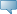# Lebanese American University WWW Information System

## Catalog Entries

Date
Sep 22, 2023Select the Course Number to get further detail on the course. Select the desired Schedule Type to find available classes for the course.

 MTH 307 - Discrete Structure II This course covers computational complexity and order analysis, recurrence relations and their solutions, graphs and trees, elementary computability, classes P and NP problems, NP-completeness (Cook’s theorem), NP-complete problems, reduction techniques, automata theory including deterministic and nondeterministic finite automata, equivalence of DFAs and NFAs, regular expressions, the pumping lemma for regular expressions, context-free grammars, Turing machines, nondeterministic Turing machines, sets and languages, uncomputable functions, the halting problem, implications of uncomputability, Chomsky hierarchy, and the Church-Turing thesis. Course Learning Outcomes: 1) Students shall be able to solve recurrence relations that may arise in determining algorithmic complexity problems. 2) Students shall be able to identify by example basic terminology associated with graphs, directed graphs, and trees. 3) Students shall be able to test a graph for the existence of an Euler path, an Euler circuit, a Hamiltonian path and a Hamiltonian circuit, and to use known graph algorithms including shortest path, spanning tree, and coloring planar graphs. 4) Students shall be able to convert between a regular language and a finite automaton that recognizes it. 5) Students shall be able to analyze algorithms complexity using O, , and Ω notations and to demonstrate an understanding of P and NP classes. 3.000 Credit hours 3.000 Lecture hours Levels: Undergraduate Schedule Types: Lecture, Tutorial Computer Science & Mathematics Department